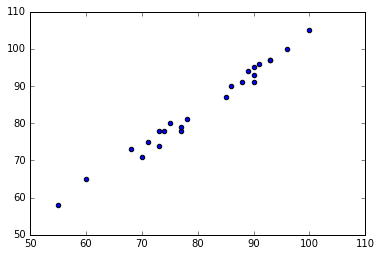• No products in the cart.

# 204.1.8 Practice : Multiple Regression Issues

##### Practicing Multi Variable Linear Regression model.

In the last post of this session, we did cover basics of Multiple variable Linear Regression. In this post, we will Practice and try to solve issues associated with Multiple Regression.

### Practice : Multiple Regression- issues

• Import Final Exam Score data
• Build a model to predict final score using the rest of the variables.
• How are Sem2_Math & Final score related? As Sem2_Math score increases, what happens to Final score?
• Remove “Sem1_Math” variable from the model and rebuild the model
• Is there any change in R square or Adj R square
• How are Sem2_Math & Final score related now? As Sem2_Math score increases, what happens to Final score?
• Draw a scatter plot between Sem1_Math & Sem2_Math
• Find the correlation between Sem1_Math & Sem2_Math
In :
#Import Final Exam Score data

In :
#Size of the data
final_exam.shape

Out:
(24, 5)
In :
#Variable names
final_exam.columns

Out:
Index(['Sem1_Science', 'Sem2_Science', 'Sem1_Math', 'Sem2_Math',
'Final_exam_marks'],
dtype='object')
In :
#Build a model to predict final score using the rest of the variables.
from sklearn.linear_model import LinearRegression
lr1 = LinearRegression()
lr1.fit(final_exam[["Sem1_Science"]+["Sem2_Science"]+["Sem1_Math"]+["Sem2_Math"]], final_exam[["Final_exam_marks"]])
predictions1 = lr1.predict(final_exam[["Sem1_Science"]+["Sem2_Science"]+["Sem1_Math"]+["Sem2_Math"]])

import statsmodels.formula.api as sm
model1 = sm.ols(formula='Final_exam_marks ~ Sem1_Science+Sem2_Science+Sem1_Math+Sem2_Math', data=final_exam)
fitted1 = model1.fit()
fitted1.summary()

Out:
Dep. Variable: R-squared: Final_exam_marks 0.990 OLS 0.987 Least Squares 452.3 Wed, 27 Jul 2016 1.50e-18 11:48:28 -38.099 24 86.20 19 92.09 4 nonrobust
coef std err t P>|t| [95.0% Conf. Int.] -1.6226 1.999 -0.812 0.427 -5.806 2.561 0.1738 0.063 2.767 0.012 0.042 0.305 0.2785 0.052 5.379 0.000 0.170 0.387 0.7890 0.197 4.002 0.001 0.376 1.202 -0.2063 0.191 -1.078 0.294 -0.607 0.194
 Omnibus: Durbin-Watson: 6.343 1.863 0.042 4.332 0.973 0.115 3.737 1200
In :
fitted1.rsquared

Out:
0.98960765475687229
• How are Sem2_Math & Final score related? As Sem2_Math score increases, what happens to Final score?

As Sem2_Math score increases Final score decreases

In :
#Remove "Sem1_Math" variable from the model and rebuild the model
from sklearn.linear_model import LinearRegression
lr2 = LinearRegression()
lr2.fit(final_exam[["Sem1_Science"]+["Sem2_Science"]+["Sem2_Math"]], final_exam[["Final_exam_marks"]])
predictions2 = lr2.predict(final_exam[["Sem1_Science"]+["Sem2_Science"]+["Sem2_Math"]])

import statsmodels.formula.api as sm
model2 = sm.ols(formula='Final_exam_marks ~ Sem1_Science+Sem2_Science+Sem2_Math', data=final_exam)
fitted2 = model2.fit()
fitted2.summary()

Out:
Dep. Variable: R-squared: Final_exam_marks 0.981 OLS 0.978 Least Squares 341.4 Wed, 27 Jul 2016 2.44e-17 11:48:29 -45.436 24 98.87 20 103.6 3 nonrobust
coef std err t P>|t| [95.0% Conf. Int.] -2.3986 2.632 -0.911 0.373 -7.889 3.092 0.2130 0.082 2.595 0.017 0.042 0.384 0.2686 0.068 3.925 0.001 0.126 0.411 0.5320 0.067 7.897 0.000 0.391 0.673
 Omnibus: Durbin-Watson: 5.869 2.424 0.053 3.793 0.864 0.15 3.898 1030
• Is there any change in R square or Adj R square
Model R2
model1 0.990 0.987
model2 0.981 0.978
• How are Sem2_Math & Final score related now? As Sem2_Math score increases, what happens to Final score?

As Sem2_Math score increases Final score also increases.

In :
#Draw a scatter plot between Sem1_Math & Sem2_Mat

import matplotlib.pyplot as plt
%matplotlib inline
plt.scatter(final_exam.Sem1_Math,final_exam.Sem2_Math)

Out:
<matplotlib.collections.PathCollection at 0xb2cf0f0>In :
#Find the correlation between Sem1_Math & Sem2_Math
np.corrcoef(final_exam.Sem1_Math,final_exam.Sem2_Math)

Out:
array([[ 1.       ,  0.9924948],
[ 0.9924948,  1.       ]])

The next post is about issues of multicollinearity in python.


Link to the next post : https://statinfer.com/204-1-9-issue-of-multicollinearity-in-python/

23rd January 2018